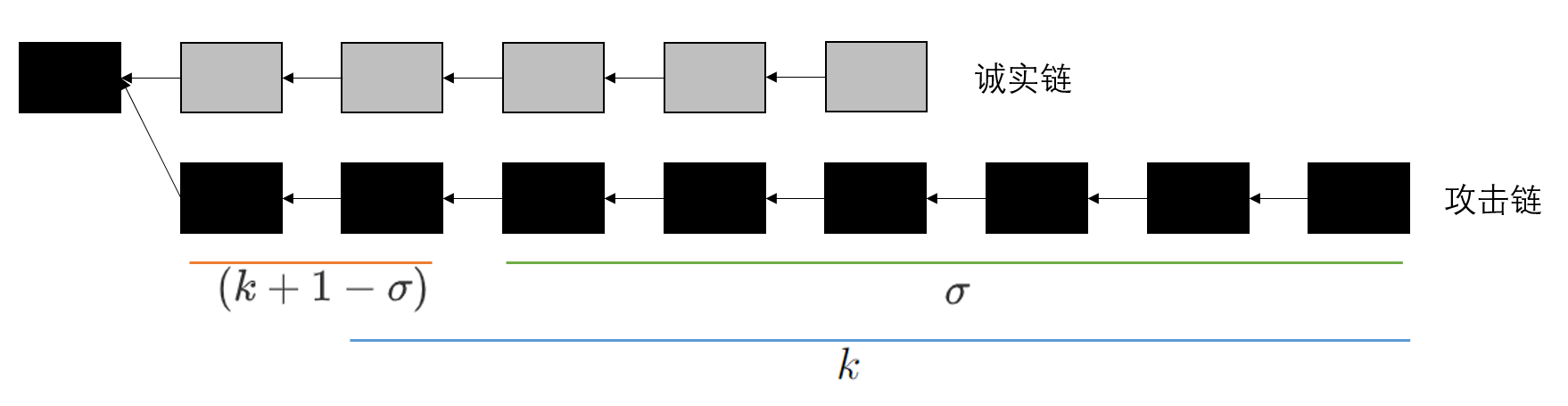# Evaluating Proof-of-Work Consensus Protocols’ Security

## 评估框架和安全模型

### Evaluation Framework

Chain quality

Incentive compatibility

Subversion gain

Subversion gain 定义为：

$\alpha$ 未双花的平均时间奖励

“作恶收益”，衡量抗双花攻击的性能，被定义为：平均每个出块间隔(能够获得的区块奖励加上双花奖励)的最大值Censorship susceptibility

$\alpha$：攻击者算力占比

$B_c/B_a$：主链上 诚实矿工/攻击矿工 的块数量

$R_c/R_a$：诚实矿工/攻击矿工 收到的奖励

$\sigma$ ：是交易确认规定的出块数量，Bitcoin中 $\sigma=6$

$k$：==被攻击者成功孤立的块数量==

$V_{ds}$ ：攻击者收到的double-spending 奖励

$t$：表示时间，单位出块时间

$O_c$：诚实矿用因为攻击存在而产生的损失